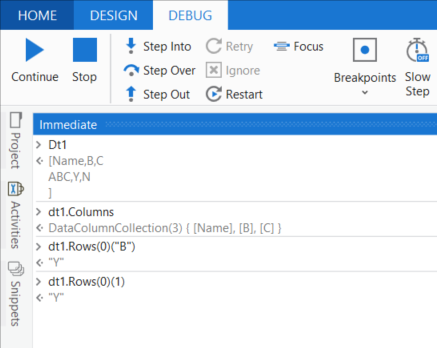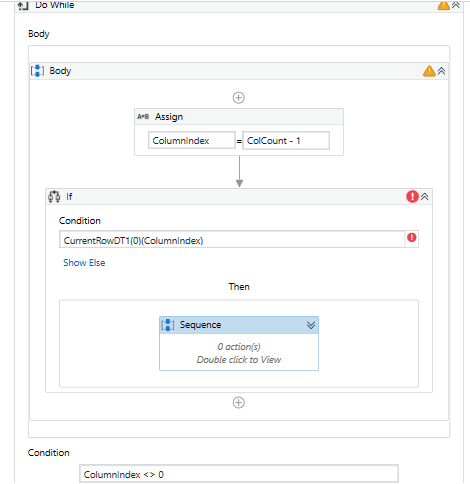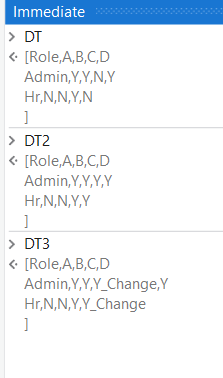# Comparison of two Datatables using two keys

Hello!

Need some help in solving issue related to comparison of two datatables.

Dummy DT1 is as below:

Role A B C D E F G H I
Administrator Y Y Y N Y Y Y
Robot N Y N N Y Y Y
Developer Y
Manager Y

Dummy DT2 is as below:

Role A B C D E F G H I
Administrator Y Y Y N Y Y Y Y
Robot N N Y N Y Y Y
Developer N N
Manager Y

Condition: If any value is not matching or is not available in any of the DTs then, Value = Value_Change
For example: DT1 - Y & DT2 - N —> Output: N_Change
DT1 - Blank & DT2 - Y —> Output: Y_Change

Issue: Not able to compare cell values using two keys. As column names cannot be predefined.
Key 1: Role
Key 2: ColumnName(A to I)

@riyatona18
Welcome to the forum

we assume that the positions will be reliable: Position B in dt1 will be the same as in dt2
So you can use the Column index instead of the column name

Hello Peter,

Yes. The column names are same in DT1 and DT2.

I’m not sure of column index as I’m new to this. How can I get that?

column index is 0 based

instead of row(“ColumnName”) - lets assume column is on 4th position we use
row(3)

Okay. Can I share xaml file here? Just to understand where am I going wrong.

find some starter helpUnderstanding the 6 Debugging Panels of UiPath in the easiest way possible! - News / Tutorials - UiPath Community Forum

What is the required output?
Can you share the output format

I’ve been trying using for each datarow activity but I don’t know how to add the if condition.

I cannot add xaml file here.

1. I’ve used two for each datarows
2. ColumnCount = DT1.Columns.Count
3. ColumnIndex = ColumnCount - 1
4. Fetching role name from both the datatables
5. Using do while till ColumnIndex <> 0
6. Using If condition. But I wonder how to add if condition.

Would it be CurrentRowDT1.item(ColumnIndex).equals(CurrentRowDT2.item(ColumnIndex))

But I’m getting error while trying this.

Attaching image:Hello @kumar.varun2

PFB input and output:

DT1

Role A B C D E F G H I
Administrator Y Y Y N Y Y Y
Robot N Y N N Y Y Y
Developer Y
Manager Y

DT2

Role A B C D E F G H I
Administrator Y Y Y N Y Y Y Y
Robot N N Y N Y Y Y
Developer N N
Manager Y

Output:

Role A B C D E F G H I
Administrator Y Y Y N Y Y Y_Change Y
Robot N N_Change Y_Change N Y Y Y
Developer N_Change N_Change
Manager Y

Please check this implementation. Not so optimized but will work.

1. Read the excel files to respective data table variables (DT1, DT2)
2. Create column name array and get the names of columns
3. Create the output data table template (Clone method)
4. Iterate through the rows and column and compare each item of both the tables.
5. Add the row to the output table
6. Write the output to excel file

DT_ComparisonTwoDataTables.zip (17.0 KB)

@riyatona18
I was working with one of my colleagues @kumar.varun2 on your case and he will provide soon an omptimized version

As informed by @ppr, the another approach is as follows. Please go through it

With the LINQ approach this can be done like this

LINQ_ComarisonTwoDataTables.xaml (7.5 KB)

The LINQ Used

``````(
From r1 In DT1.AsEnumerable
Let role = r1(0).ToString
Let ia2 = DT2.Rows(RoleIndxDict(role)).ItemArray
Let ra1 = r1.ItemArray.Skip(1).Select(Function (x, i) If(x.ToString.Equals(ia2(i+1).ToString), x, ia2(i+1).ToString & "_Change" )).ToArray
Let ra = ra1.Prepend(role).ToArray
).CopyToDataTable
``````

2 Likes

Thank you @kumar.varun2 and @ppr
I will check this approach1 Like

Thanks a lot for helping me out @kumar.varun2 & @ppr
The solution is just perfect. Much appreciated!!

1 Like